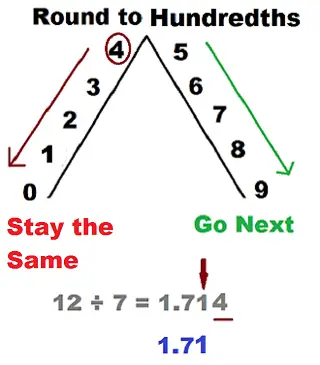# The Ultimate Rounding Decimals Worksheet

Decimals don’t need to be difficult. While we understand that some students may struggle with this topic, the truth is that we believe that it is a matter of understanding the concept.

One of the things that you may not even remember is hat you keep rounding decimals on a daily basis. No matter if you are at the grocery store and you want to ensure that you have a good idea about what you’re going to pay at the end or if you are having lunch with your co-workers and want to split the bill, you will need to round decimals.

## The Ultimate Rounding Decimals Worksheet

When you need to round decimals, you just need to keep in mind that this is a process. To round a number look at the next digit in the right place, if the digit is less than 5, round down and if the digit is 5 or more than 5, round up.

Notice that rounding decimals need to have a certain degree of accuracy. And this is why you can round decimals to the nearest wholes, tenths or hundredths.

Discover how to round to the nearest whole number.

## Rounding To The Nearest Whole

When you are trying to round decimals to the nearest whole, you just need to follow the next steps:

Step #1: Just look at the decimal that you want to round.

Step #2: When you are rounding the decimal to the nearest whole, then you need to mark the digit in the one’s place.

Looking to round to the nearest thousand?

Step #3: At this point, you will need to look at the “tenths” place which is the digit immediately to the right of the decimal point.

Step #4: Now, you have two different situations that may occur:

1- If the digit in the tenths column is 0, 1, 2, 3 or 4, you will need to round down the number at the ones place to the nearest whole number.

2- If the digit in the tenths column is 5, 6, 7, 8 or 9, you will ned to round up the number at the one’s place to the nearest whole number.

Step #5: Remove all the digits after the decimal point. The left out number is the desired answer.

Check out how to easily round to the nearest tenth.

Let’s take a look at some practical examples.

Practical Example: Let’s say that you want to round 945.65 to the nearest whole number.

So, in order to round it, you just need to follow the previous steps we described.

Step #1: 945.65 is the decimal that we want to round to the nearest whole.

Step #2: You get 5 in the one’s column.

Step #3: You get 6 in the hundredths column (right of 5).

Step #4: Since you got 6 in the previous step, then you will need to round up.

Step #5: You add 1 to 5 and remove all the digits from the right of the one’s place. So, the result will be 946.